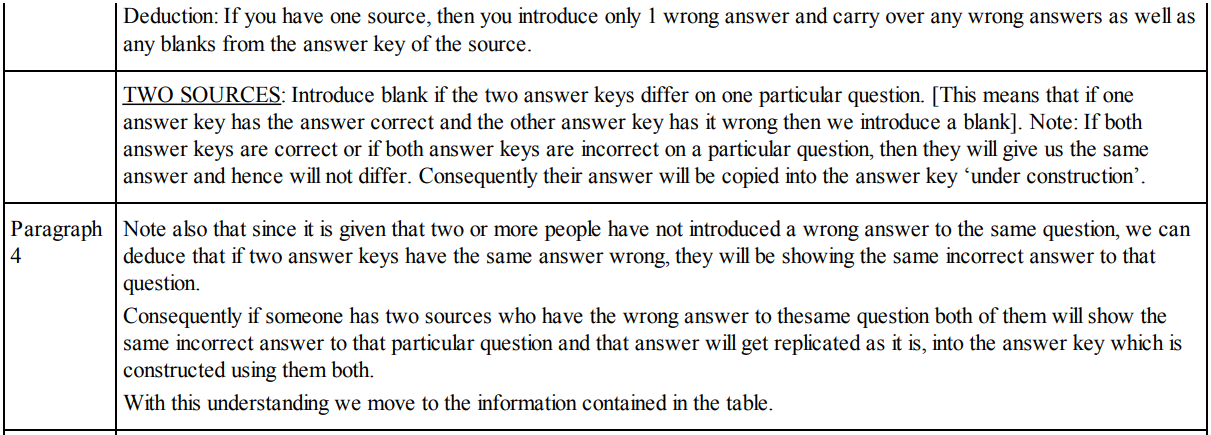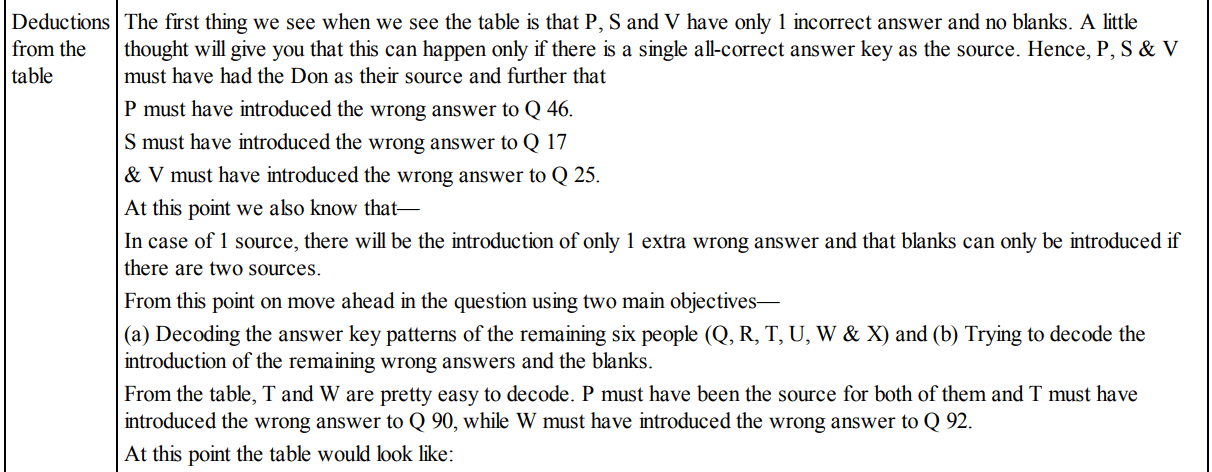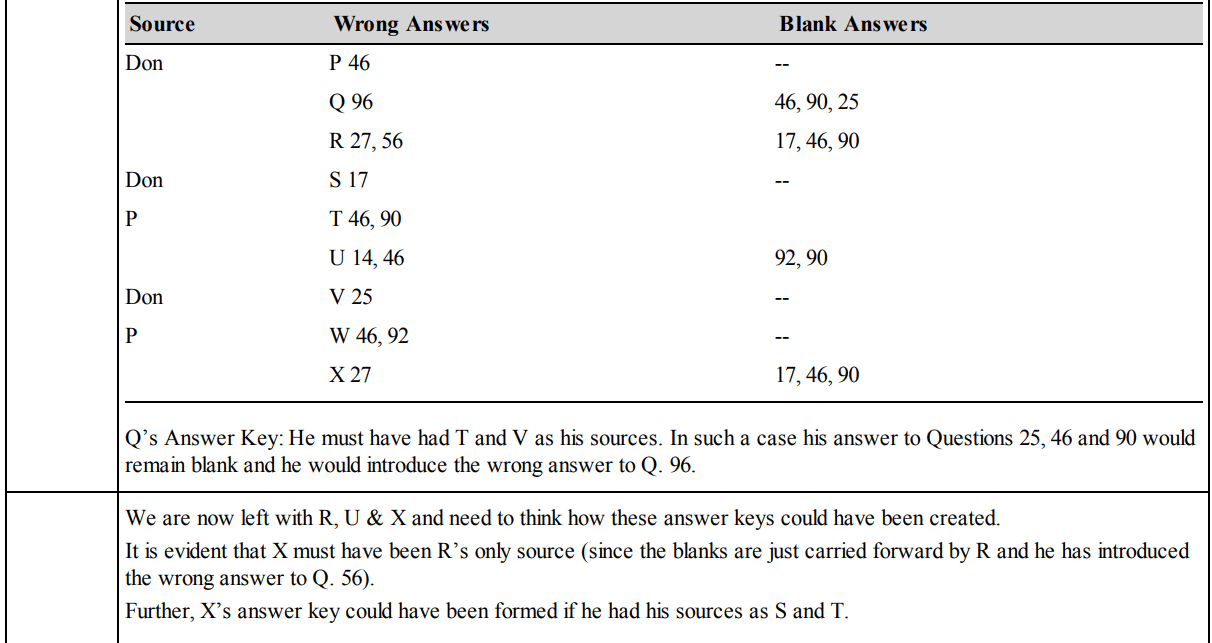## Quantitative Reasoning Questions and Answers Part-3

Data for Questions 1 to 6 : A, B, C, D and E are five different integer. When written in the ascending order of values, the difference between any two adjacent integers is 4. D is the greatest and A the least. B is greater than E but less than C. The sum of the integers is equal to E.

1. The value of A is:
a) -7
b) -9
c) -5
d) None of these

Explanation:Option (b) is correct

2. The sum of A and B is:
a) -10
b) -15
c) 10
d) None of these

Explanation: The answer is -10. Option (a) is correct (Refer explanation of Question 1)

3. The greatest number has the value:
a) 9
b) -5
c) 3
d) 7

Explanation: D is ‘7’. Option (d) is correct (Refer explanation of Question 1)

4. The sum of the integers is:
a) 25
b) -6
c) -15
d) None of these

Explanation: The value of E is –5. Option (d) is correct (Refer explanation of Question 1)

5. The product of the integers is:
a) -945
b) 945
c) 316
d) None of these

Explanation: 7 ¥ 3 ¥ 1 ¥ –5 ¥ –9 = –945. Option (a) is correct (Refer explanation of Question 1)

6. What is the positive difference between the lowest and the highest integers?
a) 8
b) 6
c) 16
d) None of these

Explanation: 7–(–9) = 16. Option (c) is correct (Refer explanation of Question 1)

Data for Questions 7 to 11 : In November the answers of a prestigious test held nationwide were leaked to a group of unscrupulous people. The CBI has arrested the Don, the mastermind behindit and nine other people—P, Q, R, S, T, U, V, W and X in this matter. On interrogation, certain facts came into light:
Their modus operandi consisted of the Don initially obtaining the answer key, then the other nine persons created their answer keys in the following manner:
They obtained the answer key from one or two sources, then he/she compares the answer keys to a question from both sources. If the key to a question from both sources is identical, it is copied, otherwise it is left blank. If the person has only one source, he/she copies the source’s answer into his/her copy. Finally, each person compulsorily replaces one of the answers (not a blank one) with a wrong answer in his/her answer key.
The paper contained 150 questions. So the CBI has ruled out the possibility of two or more of them introducing wrong answer to the same question. The CBI has a copy of correct answer key and tabulated the following data. The data represents question numbers7. Who among the following must have two sources?
a) P
b) Q
c) R
d) S

Explanation:Option (b) is correct

8. How many people (excluding the Don) needed to make answer keys before R could make his answer key?
a) 3
b) 4
c) 6
d) None of these

Explanation: P, T, S, X. Hence 4. Option (b) is correct (Refer explanation of Question 7)

9. Both T and W were sources to:
a) U
b) X
c) Q
d) None of these

Explanation: The answer is U. Option (a) is correct (Refer explanation of Question 7)

10. Which of the following is definitely true?
a) R introduced the wrong answer to question 27.
b) T introduced the wrong answer to question 46
c) U introduced the wrong answer to question 14.
d) W introduced the wrong answer to question 46.